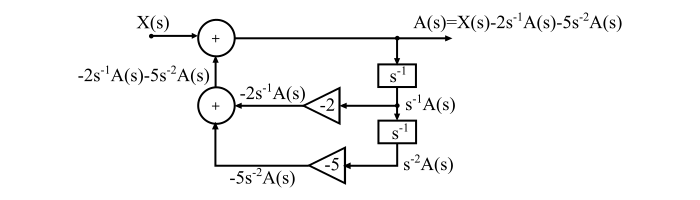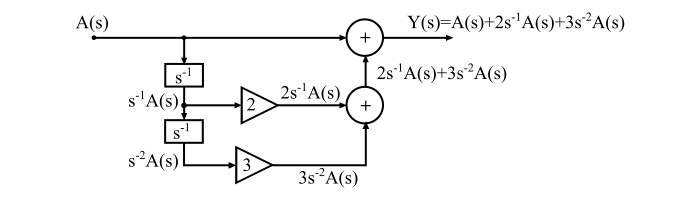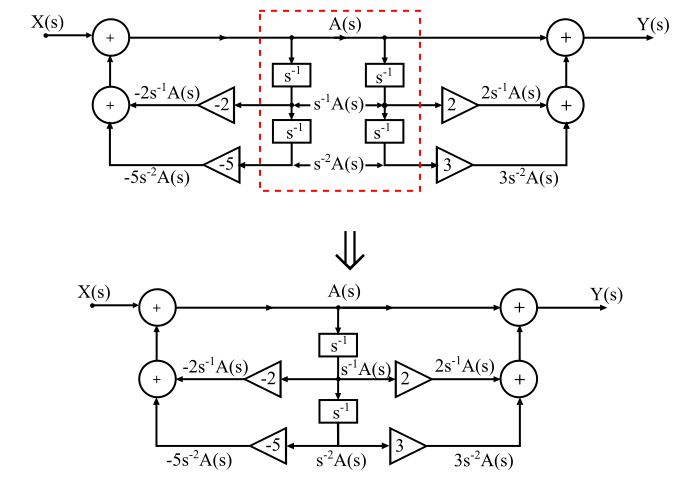# Direct Form-II Realization of Continuous-Time Systems

## Realization of Continuous-Time System

Realisation of a continuous-time LTI system means obtaining a network corresponding to the differential equation or transfer function of the system.

The transfer function of the system can be realised either by using integrators or differentiators. Due to certain drawbacks, the differentiators are not used to realise the practical systems. Therefore, only integrators are used for the realization of continuous-time systems. The adder and multipliers are other two elements which are used realise the continuous-time systems.

## Direct Form-II Realization of CT Systems

The advantage of the direct form-II realization of continuous-time systems is that it uses minimum number of integrators. In this realization structure, an intermediate variable is integrated, instead of using the separate integrators for integrating the input and output variables separately.

The direct form-II realization of continuous-time systems is explained in the following example −

## Numerical Example

Using the direct form-II, realise the continuous-time LTI system described by the following transfer function.

$$\mathrm{\mathit{H\left ( s \right )\mathrm{\,=\,}\frac{Y\left ( s \right )}{X\left ( s \right )}\mathrm{\,=\,}\frac{s^{\mathrm{2}}\mathrm{\,+\,}\mathrm{2}s\mathrm{\,+\,}\mathrm{3}}{s^{\mathrm{2}}\mathrm{\,+\,}\mathrm{2}s\mathrm{\,+\,}\mathrm{5}}}}$$

### Solution

The given function is to be expressed in the negative powers of s as −

$$\mathrm{\mathit{H\left ( s \right )\mathrm{\,=\,}\frac{Y\left ( s \right )}{X\left ( s \right )}\mathrm{\,=\,}\frac{s^{\mathrm{2}}\mathrm{\,+\,}\mathrm{2}s\mathrm{\,+\,}\mathrm{3}}{s^{\mathrm{2}}\mathrm{\,+\,}\mathrm{2}s\mathrm{\,+\,}\mathrm{5}}\mathrm{\,=\,}\frac{\mathrm{1}\mathrm{\,+\,}\mathrm{2}s^{\mathrm{-1}}\mathrm{\,+\,}\mathrm{3}s^{\mathrm{-2}}}{\mathrm{1}\mathrm{\,+\,}\mathrm{2}s^{\mathrm{-1}}\mathrm{\,+\,}\mathrm{5}s^{\mathrm{-2}}} }}$$

Let A(s) = 1, then decomposing the given transfer function into two parts as −

$$\mathrm{\mathit{H\left ( s \right )\mathrm{\,=\,}\frac{Y\left ( s \right )}{X\left ( s \right )}\mathrm{\,=\,}\frac{Y\left ( s \right )}{A\left ( s \right )}\frac{A\left ( s \right )}{X\left ( s \right )}}}$$

Where,

$$\mathrm{\mathit{\frac{Y\left ( s \right )}{A\left ( s \right )}\mathrm{\,=\,}\mathrm{1}\mathrm{\,+\,}\mathrm{2}s^{\mathrm{-1}}\mathrm{\,+\,}\mathrm{3}s^{\mathrm{-2}}\; \; \; \cdot \cdot \cdot \left ( \mathrm{1} \right )}}$$

And

$$\mathrm{\mathit{\frac{A\left ( s \right )}{X\left ( s \right )}\mathrm{\,=\,}\frac{\mathrm{1}}{\mathrm{1}\mathrm{\,+\,}\mathrm{2}s^{\mathrm{-1}}\mathrm{\,+\,}\mathrm{5}s^{\mathrm{-2}}}\; \; \; \cdot \cdot \cdot \left ( \mathrm{2} \right )}}$$

Cross multiplying the eq. (1) & eq. (2), we get,

From eq. (1),

$$\mathrm{\mathit{Y\left ( s \right )\mathrm{\,=\,}A\left ( s \right )\mathrm{\,+\,}\mathrm{2}s^{\mathrm{-1}}A\left ( s \right )\mathrm{\,+\,}\mathrm{3}s^{\mathrm{-2}}A\left ( s \right )}}$$

And from eq. (2),

$$\mathrm{\mathit{X\left ( s \right )\mathrm{\,=\,}A\left ( s \right )\mathrm{\,+\,}\mathrm{2}s^{\mathrm{-1}}A\left ( s \right )\mathrm{\,+\,}\mathrm{5}s^{\mathrm{-2}}A\left ( s \right )}}$$

$$\mathrm{\mathit{\Rightarrow A\left ( s \right )\mathrm{\,=\,}X\left ( s \right )-\mathrm{2}s^{\mathrm{-1}}A\left ( s \right )-\mathrm{5}s^{\mathrm{-2}}A\left ( s \right )}}$$

Therefore, the above transfer function can be realised as follows −

### Step 1

Realising A(s) as −### Step 2

Realising Y(s) as −### Step 3

By combining the above two realisations, we get the direct form-II realisation of the transfer function H(s) as −